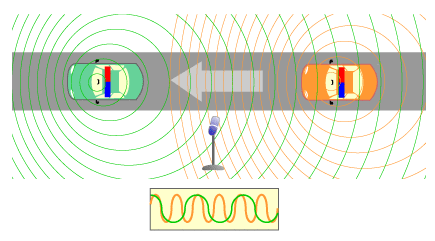homechevron_rightStudychevron_rightPhysics

# Doppler Effect in sound

Calculation of frequency and wavelength change for a moving sourceThe Doppler effect is the change in frequency of a wave for an observer moving relative to its source. It is commonly heard when a vehicle sounding a siren or horn approaches, passes, and recedes from an observer. The received frequency is higher (compared to the emitted frequency) during the approach, it is identical at the instant of passing by, and it is lower during the recession.
The relative changes in frequency can be explained as follows. When the source of the waves is moving toward the observer, each successive wave crest is emitted from a position closer to the observer than the previous wave. Therefore each wave takes slightly less time to reach the observer than the previous wave. Therefore the time between the arrival of successive wave crests at the observer is reduced, causing an increase in the frequency. While they are travelling, the distance between successive wave fronts is reduced; so the waves "bunch together". Conversely, if the source of waves is moving away from the observer, each wave is emitted from a position farther from the observer than the previous wave, so the arrival time between successive waves is increased, reducing the frequency. The distance between successive wave fronts is increased, so the waves "spread out".
Source

The Doppler effect for sound can be expressed as follows:

Frequency change

Wavelength change

For approaching source speed v' should be negative, for receding source speed v' should be positive.
v - speed of sound in the air. By default it is equal to speed of sound in the dry air at 20 degrees Centigrade, see Sound Speed in Gases#### Doppler Effect in sound

Digits after the decimal point: 2
Frequency, Hz

Observed frequency, Hz

Wavelength, m

Observed wavelength, m